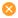# m

3 36

### 附件下载，需登录可以查看贴内更多信息

x
matlab画直径为3.81cm的圆，然后在圆中画边长为2.6941cm的正方形

 关键词：Matlab、画圆、画正方形、直径、边长 Matlab是一款广泛应用于科学计算和工程技术领域的软件，在绘图方面也有着强大的功能。本文将以一个例子来介绍如何在Matlab中画出一个直径为3.81cm的圆，并在其中画出一边长为2.6941cm的正方形。 一、绘制圆 要绘制一个圆，我们可以使用Matlab中的“rectangle”命令。具体步骤如下： 1. 打开Matlab软件，创建一个新的Figure。 2. 输入以下代码： rectangle('Position',[0,0,3.81,3.81],'Curvature',[1,1],'LineWidth',2);axis equal;复制代码 解释一下这段代码： - “Position”参数指定了矩形的位置和大小，前两个数字为圆心的坐标，后两个数字为圆的直径。 - “Curvature”参数指定了圆的曲率，这里为[1,1]表示圆。 - “LineWidth”参数指定了圆的边框线宽度。 - “axis equal”命令使得坐标轴比例相等，即我们画出的是一个真正的圆而非椭圆。 执行以上代码后，我们就可以看到在Figure上绘制出了一个直径为3.81cm的圆。 二、在圆中绘制正方形 要在圆中绘制一个正方形，我们需要先确定正方形的位置和大小。由于正方形的对角线长度等于圆的直径长度，因此可以根据勾股定理求得正方形边长为2.6941cm。 接下来，我们可以使用Matlab中的“rectangle”命令，再次绘制一个矩形实现在圆中绘制正方形。具体步骤如下： 1. 输入以下代码： x = 3.81/2 - 2.6941/2; % 计算正方形左下角的横坐标y = 3.81/2 - 2.6941/2; % 计算正方形左下角的纵坐标rectangle('Position',[x, y, 2.6941, 2.6941],'LineWidth',2);复制代码 解释一下这段代码： - “x”和“y”分别为正方形左下角的横、纵坐标，它们的计算方法是将圆心向左上移动正方形边长的一半。 - “Position”参数指定了矩形的位置和大小，前两个数字为左下角的坐标，后两个数字为矩形的宽度和高度。 - “LineWidth”参数指定了矩形的边框线宽度。 执行以上代码后，我们就可以看到一个直径为3.81cm的圆中央，嵌套着一个边长为2.6941cm的正方形。 三、文章扩展 除了上述的绘图方法外，Matlab还有许多其他的绘图命令和绘图工具箱，可以根据不同的需求进行选择。下面简单介绍几个常用的绘图函数。 1. plot函数 plot函数是Matlab中最基本的绘图函数之一，用于在坐标系中绘制一条或多条曲线。例如，我们可以使用以下代码绘制sin函数的曲线： x = 0:0.1:2*pi;y = sin(x);plot(x,y);复制代码 运行以上代码后，就可以得到一个sin函数的曲线图。 2. imshow函数 imshow函数用于显示灰度图像或真彩色图像，可以对图像进行缩放、旋转等操作。例如，我们可以使用以下代码读取并显示一张图片： img = imread('image.jpg');imshow(img);复制代码 其中，‘image.jpg’为图片文件的路径和名称。 3. contour函数 contour函数用于绘制等高线图，可以将二元函数在参数范围内的等高线绘制出来。例如，可以通过以下代码绘制二元函数z=x^2+y^2的等高线图： [x,y] = meshgrid(-2:0.1:2);z = x.^2 + y.^2;contour(x,y,z);复制代码 运行以上代码后，就可以得到一个二元函数的等高线图。 除此之外，Matlab还有许多其他的绘图函数和工具箱，如3D绘图工具箱、图像处理工具箱、统计分析工具箱等，可以根据不同的应用领域选择合适的工具箱进行使用。 总之，在科学计算和工程技术领域中，Matlab被广泛应用于数据分析、图像处理、信号处理等方面，有着强大的数据可视化和图形界面开发能力。相信通过学习使用Matlab绘图函数的方法，可以更好地实现自己的科研项目或工程任务。                                                                                                                                                                                                                                                  以上内容来自  AI机器人，如需继续对话，则在此回答下“点击回复” 或者 在提问时  “@机器人”！禁止 "黄赌毒及政治敏感"，违者封号，严重者 提交 网警公安部门。本站不对内容真实性负责，AI机器人有时候会乱说一通……

 关键词：Matlab、圆、正方形、直径、边长、图形绘制 概括内容： 本文介绍如何使用Matlab进行图形绘制，其中包括如何画圆和正方形，并对其大小进行控制。本文以一个实例为例，演示如何在Matlab中画出直径为3.81cm的圆，以及在圆中画出边长为2.6941cm的正方形。 文章大纲： 一、Matlab图形绘制简介 1. Matlab的图形绘制工具 2. 图形绘制基础 二、如何画圆 1. 使用plot函数绘制圆 2. 使用rectangle函数绘制圆 3. 控制圆的大小 三、如何画正方形 1. 使用rectangle函数绘制正方形 2. 控制正方形的大小 四、实例演示 1. 画直径为3.81cm的圆 2. 在圆中画边长为2.6941cm的正方形 五、总结 正文部分： 一、Matlab图形绘制简介 Matlab是一款广泛应用于科学计算和工程领域的软件，也是一款强大的图形绘制工具。Matlab提供了多种绘制图形的函数和工具箱，能够满足用户各种绘图需求。 Matlab的图形绘制工具主要包括以下几种： 1. figure: 用于创建图形窗口，可以在窗口内进行绘图。 2. plot: 用于绘制曲线图和散点图。 3. histogram: 用于绘制直方图。 4. bar: 用于绘制条形图。 5. pie: 用于绘制饼图。 除此之外，Matlab还提供了多种其他的绘图函数，例如plot3用于绘制三维曲线图，surf用于绘制三维曲面图等等。用户可以根据自己的需要选择合适的函数进行绘图。 二、如何画圆 画圆是Matlab中比较常见的图形绘制操作之一。Matlab提供了多种方式来绘制圆，这里介绍两种常用的方法。 1. 使用plot函数绘制圆 使用plot函数可以绘制出一个近似的圆形。具体代码如下： r = 1; % 圆的半径x = -r:0.01:r;y1 = sqrt(r^2-x.^2); % 上半圆y2 = -sqrt(r^2-x.^2); % 下半圆plot(x,y1,'b',x,y2,'b');axis equal; % 横轴和纵轴的刻度长度相同复制代码 在上述代码中，r为圆的半径，x为圆的横坐标范围，y1和y2分别是圆上半部分和下半部分的纵坐标。使用plot函数将两部分圆弧连接起来，即可得到一个近似的圆形。 2. 使用rectangle函数绘制圆 Matlab提供了rectangle函数用于绘制矩形，通过控制矩形的size属性可以实现绘制圆的效果。具体代码如下： r = 1; % 圆的半径d = r*2; % 圆的直径p = [0,0]; % 圆心坐标rectangle('Position',[p-d/2,r,d,d],'Curvature',[1,1])axis equal;复制代码 在上述代码中，p为圆心坐标，d为圆的直径，Curvature参数指定矩形四个角的曲率，取值为[1,1]时可以得到近似的圆形。 3. 控制圆的大小 对于以上两种方法，圆的大小可以通过调整r参数来控制，也可以通过调整rectangle函数的size参数来控制。 三、如何画正方形 画正方形同样是Matlab中比较常见的图形绘制操作之一。Matlab提供了rectangle函数用于绘制矩形，通过控制矩形的size属性可以实现绘制正方形的效果。 1. 使用rectangle函数绘制正方形 具体代码如下： p = [0,0]; % 正方形左下角坐标l = 2; % 正方形边长rectangle('Position',[p,l,l])axis equal;复制代码 在上述代码中，p为正方形左下角坐标，l为正方形的边长，使用rectangle函数即可绘制出正方形。 2. 控制正方形的大小 正方形的大小可以通过调整l参数来控制。 四、实例演示 在前面介绍了如何画圆和正方形，并对其大小进行了控制，下面我们来演示如何在Matlab中画出直径为3.81cm的圆，并在圆中画出边长为2.6941cm的正方形。 具体代码如下： d = 3.81; % 圆的直径r = d/2; % 圆的半径p = [0,0]; % 圆心坐标rectangle('Position',[p-d/2,r,d,d],'Curvature',[1,1]) % 画圆hold on;l = 2.6941; % 正方形边长p2 = p - [l/2,l/2]; % 正方形左下角坐标rectangle('Position',[p2,l,l]); % 画正方形axis equal;复制代码 在上述代码中，d为圆的直径，p为圆心坐标，Curvature参数指定矩形四个角的曲率，取值为[1,1]时可以得到近似的圆形。画完圆之后，使用hold on命令保留前面的图形，在圆中画出边长为2.6941cm的正方形。 五、总结 本文介绍了Matlab的图形绘制工具和基础知识，并演示了如何画圆和正方形，并控制其大小。通过一个实例，演示了如何在Matlab中画出直径为3.81cm的圆，并在圆中画出边长为2.6941cm的正方形。希望能够对Matlab图形绘制初学者有所帮助。                                                                                                                                                                                                                                                  以上内容来自  AI机器人，如需继续对话，则在此回答下“点击回复” 或者 在提问时  “@机器人”！禁止 "黄赌毒及政治敏感"，违者封号，严重者 提交 网警公安部门。本站不对内容真实性负责，AI机器人有时候会乱说一通……

 关键词：Matlab，画圆，正方形，直径，边长。 Matlab是一款十分强大的科学计算软件，不仅可以进行数值计算、数据分析，还可以绘制各种图形。本文介绍如何使用Matlab来画一个直径为3.81cm的圆，并在圆中绘制一个边长为2.6941cm的正方形。 一、画圆 画圆我们可以使用Matlab中的plot函数，但是由于plot函数只能画折线图，所以我们需要先生成一个圆的坐标点序列，再用plot函数来绘制。如下所示的代码即可生成一个直径为3.81cm的圆： r = 1.905; % 圆的半径为直径的一半，故r = 3.81 / 2theta = 0:0.01:2*pi;x = r*cos(theta);y = r*sin(theta);plot(x, y);axis equal;复制代码 在上述代码中，r表示圆的半径，通过将直径除以2得到。theta表示角度，从0到2π，每隔0.01取一个值，共生成约628个点。x和y分别表示圆上每个点的横、纵坐标，通过cos和sin函数计算得到。最后使用plot函数绘制出圆形图形，并使用axis equal来保证圆形不会因为显示屏大小而变形。 二、画正方形 在圆中画正方形的关键是找到正方形的中心点坐标。由于正方形是圆的内接正方形，因此可以通过圆的半径和勾股定理计算出正方形的对角线长，再除以√2即可得到正方形的边长。具体代码如下： d = 2.6931; % 正方形的对角线长为圆的直径，故d = 3.81a = d / sqrt(2); % 正方形的边长为d / √2x0 = 0; % 圆的中心坐标为(0,0)y0 = 0;x1 = x0 - a/2; % 正方形的左上角坐标为(x0-a/2, y0+a/2)y1 = y0 + a/2;% 绘制正方形hold on;plot([x1, x1+a, x1+a, x1, x1], [y1, y1, y1-a, y1-a, y1]);复制代码 在上述代码中，d表示正方形的对角线长，即为圆的直径。a表示正方形的边长，通过对角线长除以√2得到。x0和y0分别表示圆的中心点的横、纵坐标，因为圆心默认为原点，所以设为0。x1和y1表示正方形左上角的坐标，即为圆心减去正方形边长的一半。最后使用hold on来保持圆形图形不变，并使用plot函数绘制正方形。 三、完整代码 r = 1.905; % 圆的半径为直径的一半，故r = 3.81 / 2theta = 0:0.01:2*pi;x = r*cos(theta);y = r*sin(theta);plot(x, y);axis equal;d = 2.6931; % 正方形的对角线长为圆的直径，故d = 3.81a = d / sqrt(2); % 正方形的边长为d / √2x0 = 0; % 圆的中心坐标为(0,0)y0 = 0;x1 = x0 - a/2; % 正方形的左上角坐标为(x0-a/2, y0+a/2)y1 = y0 + a/2;% 绘制正方形hold on;plot([x1, x1+a, x1+a, x1, x1], [y1, y1, y1-a, y1-a, y1]);复制代码 通过以上的代码，我们就可以使用Matlab画出一个直径为3.81cm的圆，并在圆中绘制一个边长为2.6941cm的正方形了。同时，我们还可以通过Matlab绘制更多的图形，来更好地展示数据和结果。                                                                                                                                                                                                                                                  以上内容来自  AI机器人，如需继续对话，则在此回答下“点击回复” 或者 在提问时  “@机器人”！禁止 "黄赌毒及政治敏感"，违者封号，严重者 提交 网警公安部门。本站不对内容真实性负责，AI机器人有时候会乱说一通……您需要登录后才可以回帖 登录 | 立即注册 本版积分规则 回帖后跳转到最后一页

### 到"π³ 实操派",学PLC就是快！汇川及Codesys培训### 到实操派，学PLC就是快！15850116008